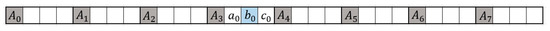Previous Article in Journal
Mathematical Properties on the Hyperbolicity of Interval Graphs
Open AccessArticle

# Discrete Sine Transform-Based Interpolation Filter for Video Compression

byMyungJun Kim andYung-Lyul Lee *Department of Computer Engineering, Sejong University, Seoul 05006, Korea
*
Author to whom correspondence should be addressed.
Symmetry 2017, 9(11), 257; https://doi.org/10.3390/sym9110257
Received: 14 October 2017 / Revised: 30 October 2017 / Accepted: 30 October 2017 / Published: 2 November 2017
Fractional pixel motion compensation in high-efficiency video coding (HEVC) uses an 8-point filter and a 7-point filter, which are based on the discrete cosine transform (DCT), for the 1/2-pixel and 1/4-pixel interpolations, respectively. In this paper, discrete sine transform (DST)-based interpolation filters (DST-IFs) are proposed for fractional pixel motion compensation in terms of coding efficiency improvement. Firstly, a performance of the DST-based interpolation filters (DST-IFs) using 8-point and 7-point filters for the 1/2-pixel and 1/4-pixel interpolations is compared with that of the DCT-based IFs (DCT-IFs) using 8-point and 7-point filters for the 1/2-pixel and 1/4-pixel interpolations, respectively, for fractional pixel motion compensation. Finally, the DST-IFs using 12-point and 11-point filters for the 1/2-pixel and 1/4-pixel interpolations, respectively, are proposed only for bi-directional motion compensation in terms of the coding efficiency. The 8-point and 7-point DST-IF methods showed average Bjøntegaard Delta (BD)-rate reductions of 0.7% and 0.3% in the random access (RA) and low delay B (LDB) configurations, respectively, in HEVC. The 12-point and 11-point DST-IF methods showed average BD-rate reductions of 1.4% and 1.2% in the RA and LDB configurations for the Luma component, respectively, in HEVC. View Full-Text
Show FiguresFigure 1

MDPI and ACS Style

Kim, M.; Lee, Y.-L. Discrete Sine Transform-Based Interpolation Filter for Video Compression. Symmetry 2017, 9, 257.